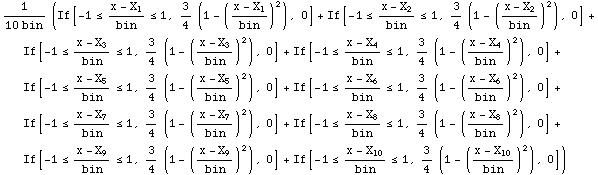### Non-Parametric Kernel Density Estimation

Example 1:    Kernel density estimation

Here is some raw data measuring the diagonal length of 100 forged Swiss bank notes and 100 real Swiss bank notes (Simonoff, 1996):

``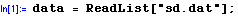``

Non-parametric kernel density estimation involves two components:
(i)  the choice of a kernel, and (ii) the selection of a bandwidth.

Here we use a Gaussian kernel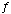:

``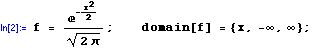``

Next, we select the bandwidth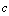. Small values for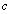produce a rough estimate while large values produce a very smooth estimate. A number of methods exist to automate bandwidth choice; mathStatica implements both the Silverman (1986) approach and the more sophisticated Sheather and Jones (1991) method. For the Swiss bank note data set, the Sheather--Jones optimal bandwidth (using the Gaussian kernel) is:

``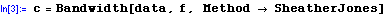``
`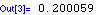`

We can now plot the smoothed non-parametric kernel density estimate using the NPKDEPlot[data, kernel, c] function:

``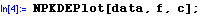``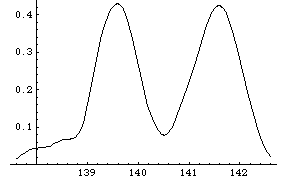Fig. 1: The smoothed non-parametric kernel density estimate (Swiss bank notes)

Example 2:    Family of CurvesInstead of presenting a single curve estimate corresponding to a single bandwidth, Marron and Chung (2001) argue that it is beneficial to present a family of curves corresponding to a range of different bandwidths. The family of curves reveals deeper structure, can show more information in a single plot, and can make it easier to select an appropriate bandwidth.

To illustrate, we consider Parzen’s (1979) yearly ‘Snowfall in Buffalo’ data (63 data points collected from 1910 to 1972, and measured in inches):

In:=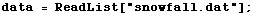Kernel: We shall use an Epanechnikov kernelhere:

In:=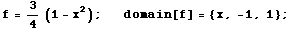Bandwidth: We shall select qq = 11 different bandwidths, ranging from a minimum (bumpy) to a maximum (smooth), as follows:

In:=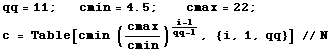Out:=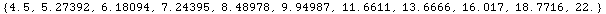Then, in mathStatica 1.2, the selected family of curves is neatly obtained with:

In:=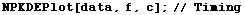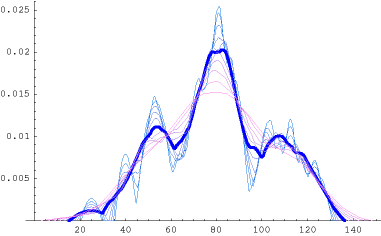Out:=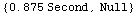References

Marron, J.S. and Chung, S.S. (2001), Presentation of smoothers: the family approach, Computational Statistics, 16, 195-207.

Non-parametric kernel density estimation is highly computationally intensive. Consequently, some computer programs try to resolve the speed problem by computing the kernel density estimate using approximate / inexact methods that reduce the amount of computation involved. Such methods do not calculate the desired estimate per se; rather, they provide an approximation of the estimate. By contrast, mathStatica always uses exact methods, and so tries to tackle the speed problem by using carefully optimised code.

To illustrate that mathStatica's NPKDE function calculates the kernel density estimate using exact methods, we now provide a completely algebraic / symbolic example. Suppose we select an Epanechnikov kernel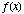:

In:=... and that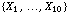is a random sample of size n = 10:

In:=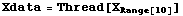Out:=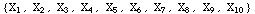Then, for any arbitrary bandwidth bin, the symbolic non-parametric kernel density estimator, calculated at an arbitrary point x, is:

In:=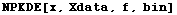Out:=